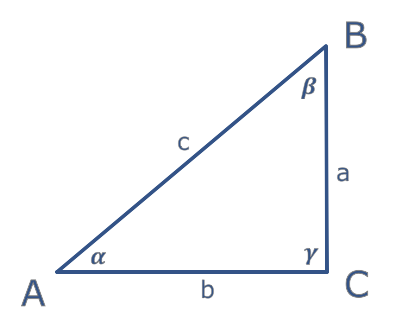# A Xojo issue or I'm not writing my code properly?

Hello all.

This may sound like a basic math problem for some, but I’m really bad in trigonometry and “visual” mathematics (a.k.a geometry).
I need to compute the hypotenuse and one side of a right triangle, knowing the angle (in degrees) and the non-hypotenuse side’s length linked to that angle.

So I made this test code, in a TextField’s TextChange event, to test whether I got it right (I didn’t):

``````dim Angle As Double=val(me.Text)*3.1415926535/180 //Convert to radian
dim hy As Double=100/sin(Angle) //100 being the known side's length; should return the hypotenuse length
dim co As Double=100*tan(Angle) //should return the other side's length

LblResult.Text="hy="+str(hy)+EndOfLine+"co="+str(co)+EndOfLine+"Angle="+str(Angle)
``````

When I input 45, the values seem right, but as more as I increase the input, the more the answer is wrong (for 90 degrees, I’m getting a side of 2.227341e+12, which is clearly wrong; for 80 degrees, I get 567.12).

I’ve checked on some websites, but they seem to use the same formula; so I’m wondering if it’s a Xojo issue or if I did the same mistake as the websites I visited…

An idea?

at 90° the tan is infinite, aka xxxe+12 result is “normal”
it simply doesn’t exists.

Ok, that makes sense then.
But how am I supposed to know the actual length of the side if the function returns infinite? Another function available?
And why is it also wrong for 80 (and worse when the value increases)?

Thank you.

tan(80°)~ 5.6712
so times 100 the result is ok.
if you have an angle of 90°, then the opposite length of the triangle is really infinite !
there is no function for that
just have a if test to check the angle and answer nothing if it is 90°

A triangle with a side of 100 in length and an angle of 80 can have another side being 567 in length? I fail to understand how this is a correct result.

I’ll do that. Thank you.

Why not? It looks like this:

100 left side 567 base

Tan(80 deg) is NOT 5.67 the Tan(80 RAD) is however

make sure you use the correct units or you will be severly messed up

Tan(80 deg) is 5.67, maybe you are confused with Excel where the Tan function needs a number in Radians, so first you convert the degrees to radians and then use tan, like: =tan(radians(80))

you are correct… seems the DEG/RAD indicator on the macOS Calculator is either backwards or very misleading

Oh yes, I see. This is possible.

But there’s still something wrong in my test code (see my first post):
For a rectangle, with these angles (90, 80 and 110), and one side being 100, how can the other two sides be 101.54 and 567.12 (i.e. the values my code returns)?

Thank you.

Yes. I wish all these “duplicates” units would disappear and we always use the same everywhere (like the ISO standard).
Complicating things for the sake of history…Thank you.

A “rectangle” has interior angles of 360 degrees (a square for example has 4 90deg corners), as such the length of the opposite sides can be any value, the only restriction is that opposite sides are equal… A Trapazoid has 4 sides but not equal sides.

A Triangle has stricter rules… its interior angles are 180deg (hence 90, 80 and 10) which match the example that Norman provided

Thanks.
Actually, I know this.

But the three sides of a right triangle cannot be 100, 101 and 567, can they?

No.

You can change your formula from:

``````dim hy As Double=100/sin(Angle)
``````

to

``````dim hy As Double=100/cos(Angle)
``````

Edit: if you have one side and the angle you either use cos/tan or sin/cot for your formulas if you want to use the same angle.

I think the problem here stems in part from not properly specifying what you have:

To illustrate, the naming convention in a triangle where I come from is:A triangle is defined by three points A,B,C that do not lie on a line.
Side a is opposite of point A, side b is opposite of point B, side c is opposite of point C.
alpha is the angle at point A and opposite side a … etc

So assuming a is the side that you know the length of:

Or do you mean beta, the angle adjacent to a?

Note that the sum of the angles in a triangle is 180 degree.
As gamma is 90 degree, beta = 90 - alpha

### Right Scalene Triangle

Side a = 575.87705
Side b = 100
Side c = 567.12818

Angle ∠A = 90° = 1.5708 rad = π/2
Angle ∠B = 10° = 0.17453 rad = π/18
Angle ∠C = 80° = 1.39626 rad = 4/9π

So you understand my question.

I’ll try, thanks.

That’s certainly true. I know the terms in French and I tried somehow to describe them in English.

In the triangle you’re showing, I know the three angles and b (adjacent to α) and I need to calculate a (the opposite side) and c (the hypotenuse).

Thank you.

Looks like the formula I have for the hypotenuse is the wrong one, then.
Thanks.

What is easy to understand is that the sum of any two sides of a triangle must be larger than the third (just draw one long base and two short arms - the arms will never be able to meet to complete the triangle)…

This is not the case for “But the three sides of a right triangle cannot be 100, 101 and 567, can they?”, so the answer is No.